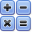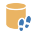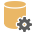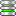##Offline Formulas

Formulas are saved in OLJ and OLB files.To create a column formula, click Column in the Formula tab. You can set a column formula to be the default value for the column.

Different formulas have different names. For example, the formula for cell B10 is named FormulaCellB10.

Function FormulaCellB10(nCol, nRow)
FormulaCellB10 = "Value"
End Function

The global formula function is named Formula.

Formulas return "Value" by default. You can return a different value by setting FormulaName=myvalue.

All formulas are sent nCol and nRow parameters.

### CELL VALUES

You can access other cells with Grid.Cell(nCol, nRow). For example, the cell to the left is:

Grid.Cell(nCol-1, nRow)

You can also access columns by name. For example:

Grid.Cell("Customer", nRow)

gets the Customer value in the same row.You can click Type in the Formula tab to change formula types (for example: from a cell formula to a column formula). You can also remove a formula. If you convert a formula to None it is calculated once and then converted to text.

When formulas are active FX displays in the status bar.

### LANGUAGE FUNCTIONS

Functions such as date and time are provided by the VBScript language. For example:
Function FormulaColTimestamp(nCol, nRow)
FormulaColTimestamp = date & " " & time
End Function
displays date and time.

### TOTALS and AVERAGES

You can access any number of cells in your (aggregate) function using for-next loops.

For example, the following cell formula (A10) calculates a total from the cells above it:

Function FormulaCellA10(nCol, nRow)
fTotal=0.0
For nr = 1 To nRow - 1
On Error Resume Next 'ignore non-numbers
fTotal=fTotal+CDbl(grid.CellText(nCol,nr))
Next
FormulaCellA10=fTotal 'modify to match the formula name if not A10
End Function

If you wanted an average instead of a total replace FormulaCellA10=fTotal with FormulaCellA10=fTotal/(nRow-1):You cannot use Grid.Cell to read a cell that is itself a formula. Instead, call the formula function for that cell directly. If you attempt to read a formula cell you will get a Script reentry evaluating cell error.

You can dowload a sample here: formulagrandtotal.olz.

### DISPLAY and UPDATE

Formulas are calculated as they are displayed on the screen.

If you have a very large Offline document (for example: 100,000 rows) only the formulas that you see on the screen are calculated.

However if you convert a formula to text, the entire column is first calculated. This allows you to disable a formula but keep the value.

All rows are are also calculated if you synch Write to a data file or server. This allows you to update a data column from a formula.SQL Offline © 2014 Interscape Corporation